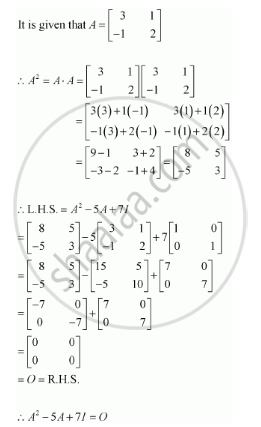Share

# If a = [(3,1),(-1,2)] Show that Asqrt2 - 5a + 7i = O - CBSE (Commerce) Class 12 - Mathematics

ConceptOperations on Matrices Properties of Multiplication of Matrices

#### Question

if A = [(3,1),(-1,2)]  show that  A^2 - 5A + 7I = O

#### SolutionIs there an error in this question or solution?

#### Video TutorialsVIEW ALL 

Solution If a = [(3,1),(-1,2)] Show that Asqrt2 - 5a + 7i = O Concept: Operations on Matrices - Properties of Multiplication of Matrices.
S